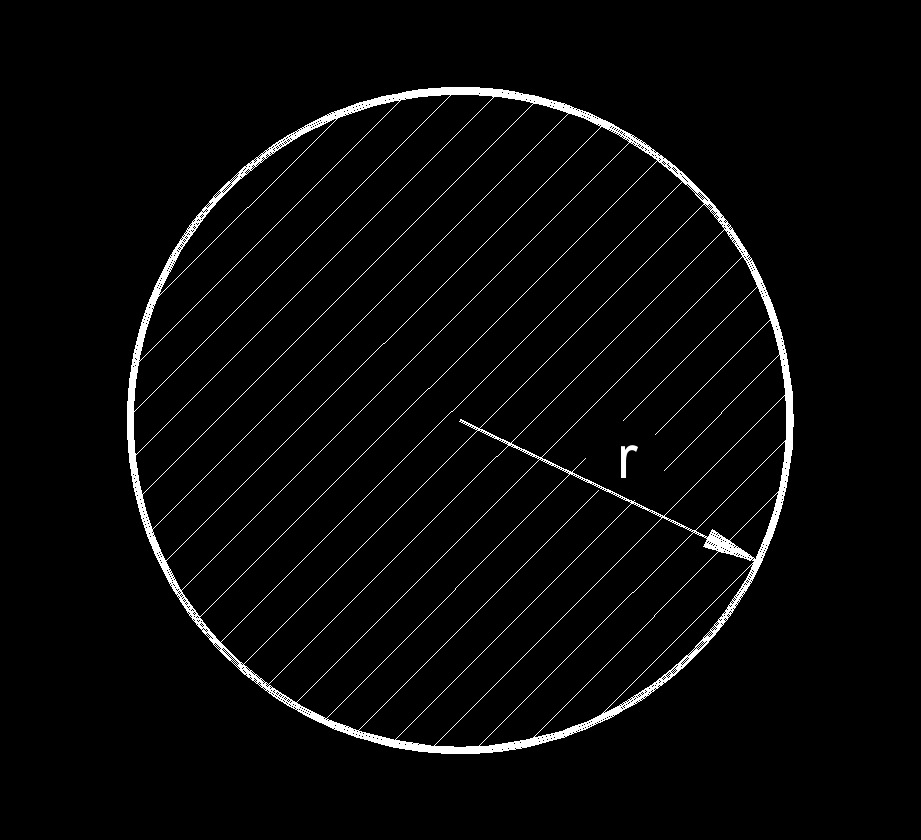# Hydraulic Diameter of a Duct, Pipe or Tube

Written by Jerry Ratzlaff on . Posted in Fluid DynamicsThe hydraulic diameter, abbreviated as Dh, is used to calculate the Reynolds Number, friction factor or for calculating pressure drop across two points.  For most geometries, the hydraulic diameter, or characteristic length, is not equal to the diameter of the shape.  However, in a circular application such as a pipe, duct or tube, the hydraulic diameter is equal to the diameter.

 $$\large{ d_h = \frac { 4 \; \pi \; r^2 } { 2 \; \pi \; r } }$$ Where: $$\large{ d_h }$$ = hydraulic diameter $$\large{ r }$$ = pipe inside radius